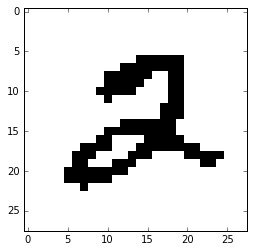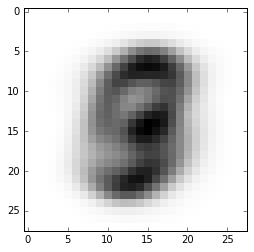In :
from matplotlib import pyplot as plt
import numpy as np
%matplotlib inline


# Self-Conjugacy for the Mean Parameter¶

\begin{align} p(\mu | x,\alpha, \sigma^2, \gamma^2) &= p(x| \mu, \alpha, \sigma^2, \gamma^2)p(\mu| \alpha, \gamma^2) / Z \qquad\text{(Bayes rule)}\\ &= p(x| \mu, \sigma^2)p(\mu| \alpha, \gamma^2) / Z \qquad\text{(Conditional independence)}\\ &\propto p(x| \mu, \sigma^2)p(\mu| \alpha, \gamma^2) \qquad\text{(Dropping the }Z)\\ &\propto \exp\left(-\frac{(x - \mu)^2}{2\sigma^2}\right)\exp\left(-\frac{(\mu - \alpha)^2}{2\gamma^2}\right) \qquad\text{(Subbing in the expression for the pdf and dropping constants)}\\ &= \exp\left(-\frac{(x - \mu)^2}{2\sigma^2}-\frac{(\mu - \alpha)^2}{2\gamma^2}\right) \end{align}

From this point it's just algebra (with some completing the square) to manipulate this into the form: $$\exp\left(-\frac{(x - a)^2}{2b^2}\right)$$ For some $a$ and $b$ (which you need to find!). That implies that $p(\mu | x,\alpha, \sigma^2, \gamma^2)\sim \mathcal{N}(a, b)$

# EM for Semi-supervised learning¶

We began by going through Section 2.1 of Mark's notes on EM for semi-supervised learning. There's a lot going on, so I'd encourage you to go through them again.

Those notes show that our E-step requires us to compute: $$r_0^i = p(\tilde{y}^i = 0|\tilde{x}_i, \theta_t)\qquad\text{and}\qquad r_1^i = p(\tilde{y}^i = 1|\tilde{x}_i, \theta_t)$$

From question 2, we have that: \begin{align} p(\tilde{y}^i = 0|\tilde{x}_i, \theta_t) &= p(x = \tilde{x}_i|y=0,\mu^0_t, \Sigma^0_t) * p(y=0|\theta_t) / Z\\ &= MVN(x=\tilde{x}_i|\mu^0_t, \Sigma^0_t) * \theta^0_t/ Z \end{align} Where MVN is the pdf of the multivariate normal. So we can calculate $r_0^i$ and $r_1^i$ by just plugging our parameters at iteration $t$ into the formulae we have and then re-normalizing.

For the M-step, we have to derive the maximum likelihood estimates for our parameters from the following equation (from Mark's notes): $$Q(\theta|\theta_t) = \sum_{i=1}^n \log p(y_i ,x_i |\theta)+ \sum_{i=1}^t\sum_{\tilde{y}_i\in\{0,1\}} r_{\tilde{y}_i}^i\log p(\tilde{y}_i ,\tilde{x}_i |\theta).$$ So, for example, we'd solve for $\mu_0$ in $\nabla_{\mu_0}Q(\theta|\theta_t) = 0$.

Now, notice that this is very simmilar to what we had to do in question 2. The first term is exactly the same as what we had previously, at the second term (the sum over $t$) is just a weighted sum of what we had before where we imagine that $\tilde{y}_i = c$. So using what we did last week, we have: \begin{align} \nabla_{\mu_0} Q(\theta|\theta_t) &= \sum_{i=1}^n \mathbb{I}(y_i = 0)\Sigma_0^{-1}(x_i - \mu_0) + \sum_{i=1}^t\sum_{\tilde{y}_i\in\{0,1\}} r_{\tilde{y}_i}^i \mathbb{I}(y_i = 0)\Sigma_0^{-1}(x_i - \mu_0).\\ &= \sum_{i=1}^n \mathbb{I}(y_i = 0)\Sigma_0^{-1}(x_i - \mu_0) + \sum_{i=1}^t r_{0}^i\Sigma_0^{-1}(x_i - \mu_0).\\ \end{align} Setting this equal to zero we get: \begin{align} \sum_{i=1}^n \mathbb{I}(y_i = 0)\Sigma_0^{-1}(x_i - \mu_0) &+ \sum_{i=1}^t r_{0}^i\Sigma_0^{-1}(x_i - \mu_0) = 0\\ \sum_{i=1}^n \mathbb{I}(y_i = 0)x_i + \sum_{i=1}^t r_{0}^i x_i & = \mu_0 \sum_{i=1}^n \mathbb{I}(y_i = 0) + \sum_{i=1}^t r_{0}^i\mu_0 \\ \mu_0 &= \frac{1}{n_0 + r_{0}} ( \sum_{i=1}^n \mathbb{I}(y_i = 0)x_i + \sum_{i=1}^t r_{0}^i x_i ) \\ \end{align} where $r_{0} = \sum_{i=1}^t r_{0}^i$. Notice that this formula makes sense, because the $r_{0}^i$ act as a sort of "soft" indicator function.

You can derive the rest of the parameters similarly.

# Independent Bernoullis for MNIST¶

In :
from keras.datasets import mnist # you can download MNIST from the course website, but I had this package available
(X_train, y_train), (X_test, y_test) = mnist.load_data()

In :
def binarize(X):
# Convert to 0 / 1 values
return 1.*(X/255. > 0.5)
X_train = binarize(X_train)
X_test = binarize(X_test)

In :
plt.imshow(X_train[5.,:], cmap='Greys',  interpolation='nearest')

/Users/jasonhar/anaconda/lib/python2.7/site-packages/ipykernel/__main__.py:1: VisibleDeprecationWarning: using a non-integer number instead of an integer will result in an error in the future
if __name__ == '__main__':

Out:
<matplotlib.image.AxesImage at 0x10b91a690>In :
n_1 = X_train.sum(axis=0) # get parameter estimates for 1s
n_0 = (1-X_train).sum(axis=0) # get parameter estimates for 1s
theta = n_1 / (n_0 + n_1) # p(x = 1 | theta)

In :
plt.imshow(theta, cmap='Greys',  interpolation='nearest')

Out:
<matplotlib.image.AxesImage at 0x10ba09a50>In :
def nll(X, theta):
return -(X * np.log(theta)[None,:,:]).sum()

In :
nll(X_test, theta) # we get a nan because there are numbers where we get 0 * -inf = nan

Out:
nan
In :
theta_lap = (n_1+1) / ((n_0+1) + (n_1+1))

In :
plt.imshow(theta_lap, cmap='Greys',  interpolation='nearest')

Out:
<matplotlib.image.AxesImage at 0x10f22df50>In :
nll(X_test, theta_lap) / X_test.shape # I'm dividing by the number of test examples to get average nll.

Out:
124.3428294047267

Notice that the laplace smoothing means we no longer the a nan for our NLL... but our density model still isn't great! You'll have to do the conditional density version to clean things up...

# Mixture of experts and mixture density networks¶

We talked briefly about mixture of experts models (which you can fit via EM) and mixture density networks (which you fit by just optimizing the likelihood function). These models treat the parameters of a mixture of gaussian model, $\pi, \mu$ and $\sigma$ as functions of some features in your data, so we have $\pi(x_i), \mu(x_i)$ and $\sigma(x_i)$. This allows you to use mixtures of gaussians for supervised density estimation.

For a nice discussion of mixture of experts models, see either Murphy or Chris Bishop's Pattern Recognition and Machine Learning. Bishop also has a good discussion on Mixture density network (he's the author of the original paper on the topic).

Alex Graves generated renewed interest in mixture density networks by using them for his handwriting models that use a recurrent network to output $\pi(x_i), \mu(x_i)$ and $\sigma(x_i)$. These models are nice flexible approaches to modeling continuous distributions without having to discritize them.

In [ ]: